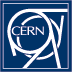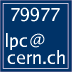Luminosity Calculator## Sources for the values in the presets

Bunch population, number of bunches and colliding bunches, emittance, beta* and the corresponding crossing angles in the experiments are taken from the talk of Run 3 Configuration WG report. The process cross section of 80mb is the agreed value established by the LPC to allow for comparision of pileup values.

The separations in ALICE and LHCb have been chosen such that the interaction rate in ALICE is close to 500 kHz and that the luminosity in LHCb is close to 2E34.

 Select a preset : Disclaimer INPUT: OUTPUT: Lorentz factor γ = γ = E / m Transverse emittance ε = m ε = εN / γ Transverve beam size at IP σx = σy = μm σx = σy = (ε β*)1/2 Effective area x-size at IP Σx = μm Σx2 = 2σx2cos2(α/2) + 2σz2sin2(α/2) Effective area y-size at IP Σy = μm Σy2 = 2σy2 Geometric factor S = S = 21/2 σx / Σx Separation factor F = F = exp(-d2 / 2Σy2 ) Luminosity per bunch pair Lbb = Hz/cm2 Lbb = frev N2 cos2(α/2) F / (2πΣxΣy) = frev N2 cos2(α/2) S F / (4πσxσy) Avg interactions per crossing μ = μ = σproc Lbb / frev Luminosity (all bunches) L = Hz/cm2 L = nbb Lbb Integrated luminosity Lint = pb-1 Lint = L H T Rate of processes R = Hz R = L σproc Lumi half life (burn-off 1 IP) τ = h τ = (1 h / 3600 s) (21/2-1) N / (Lbb σproc) Stored beam energy Estored = MJ Estored = E N kb Transverse beam spot σxytrans = μm σxytrans = σx / 21/2   (correct for head-on colliding bunches) Longitudinal beam spot σzlong = cm σzlong = σz / 21/2 * S   (correct for head-on colliding bunches)

For non-equal beta star and emittance values, the luminosity formula (for zero crossing angle) is Lbb = frev N2 / {2π (εx1β*x1x2β*x2)1/2y1β*y1y2β*y2)1/2}  , where the indices 1,2 are for the beam and x,y for the plane   (   σij = (εijβ*ij)1/2  ).

For the burn-off half-life one must give the value of the total cross section in σproc